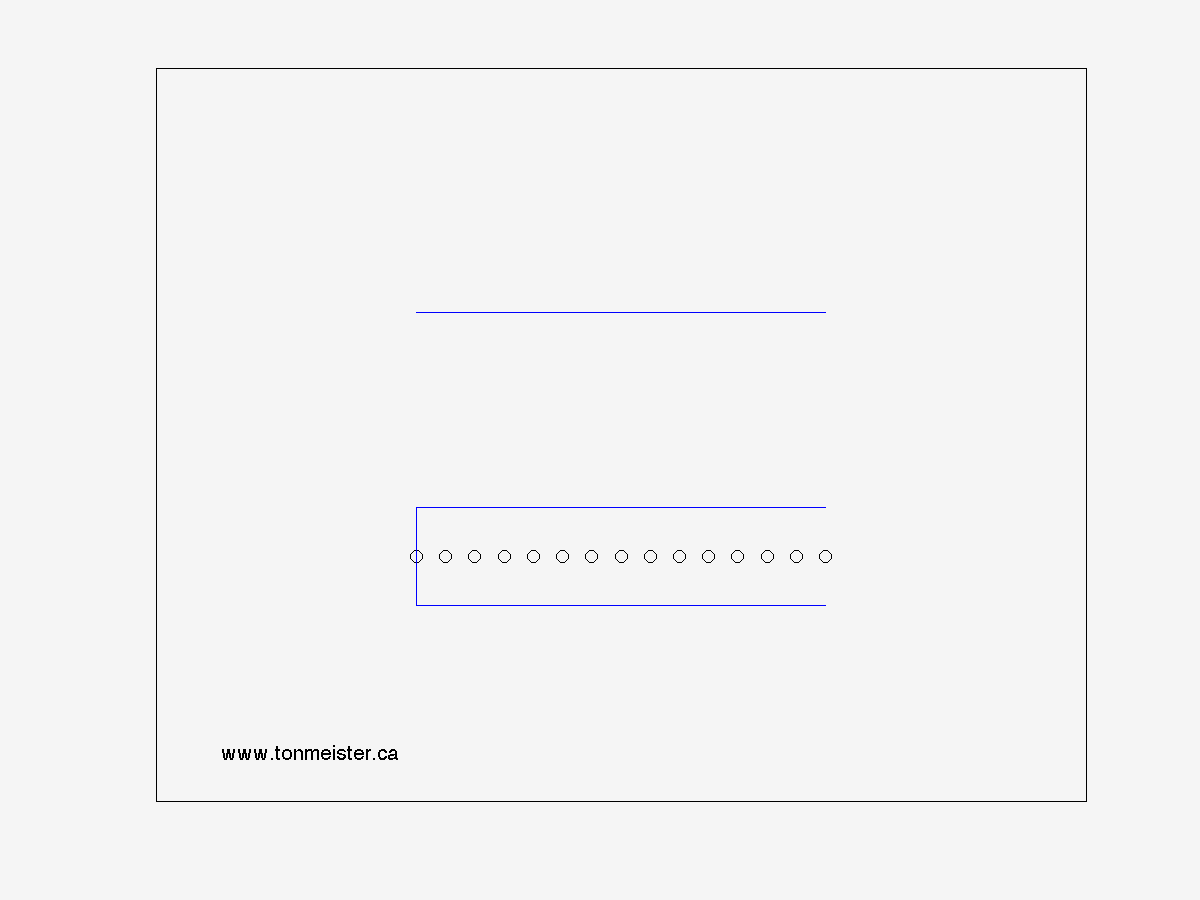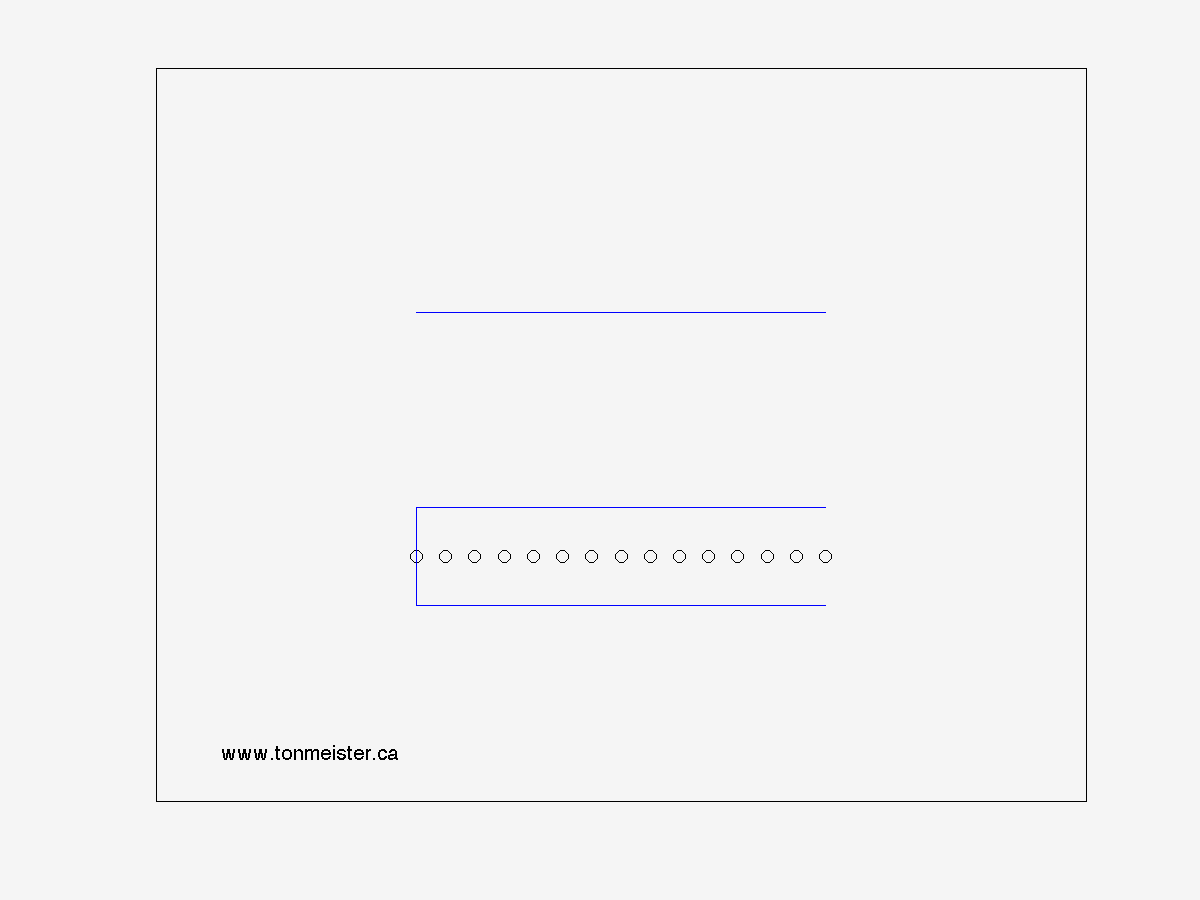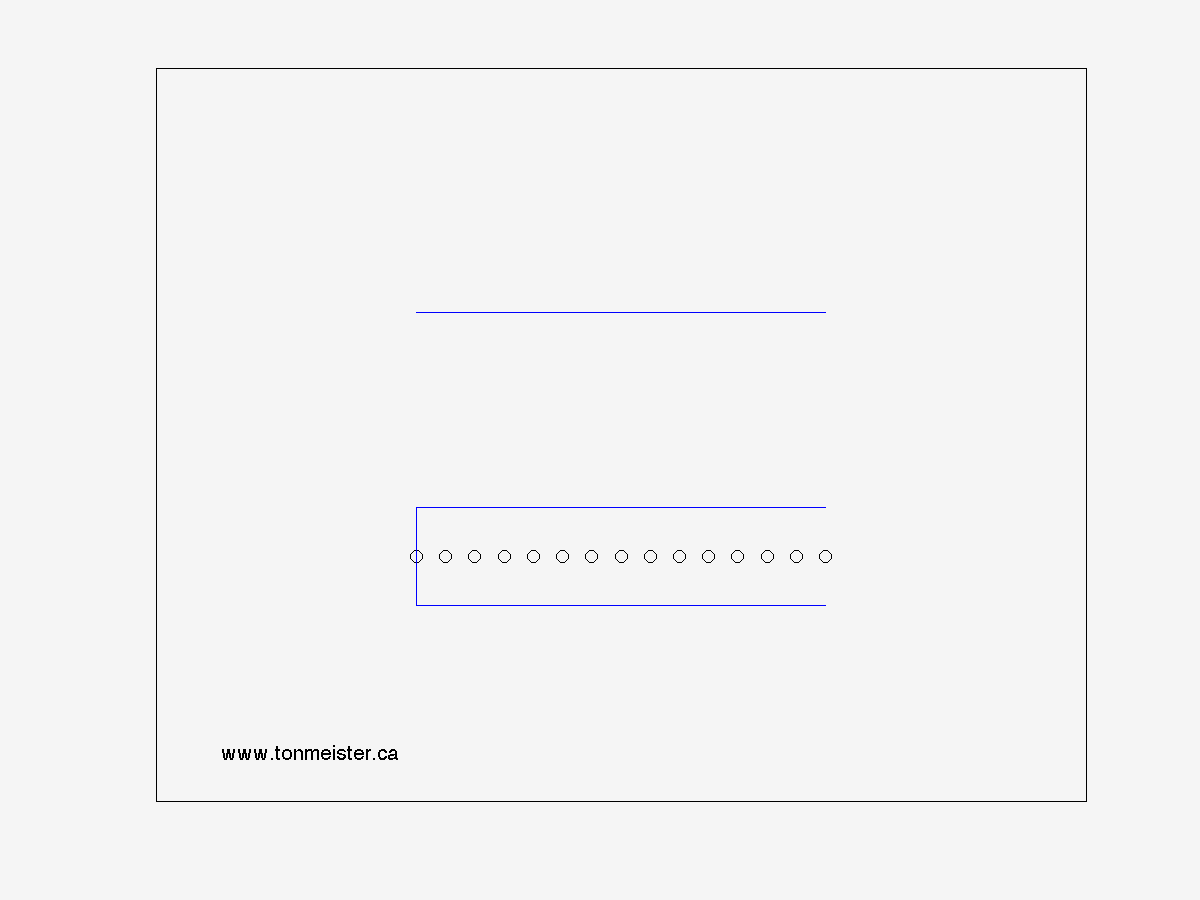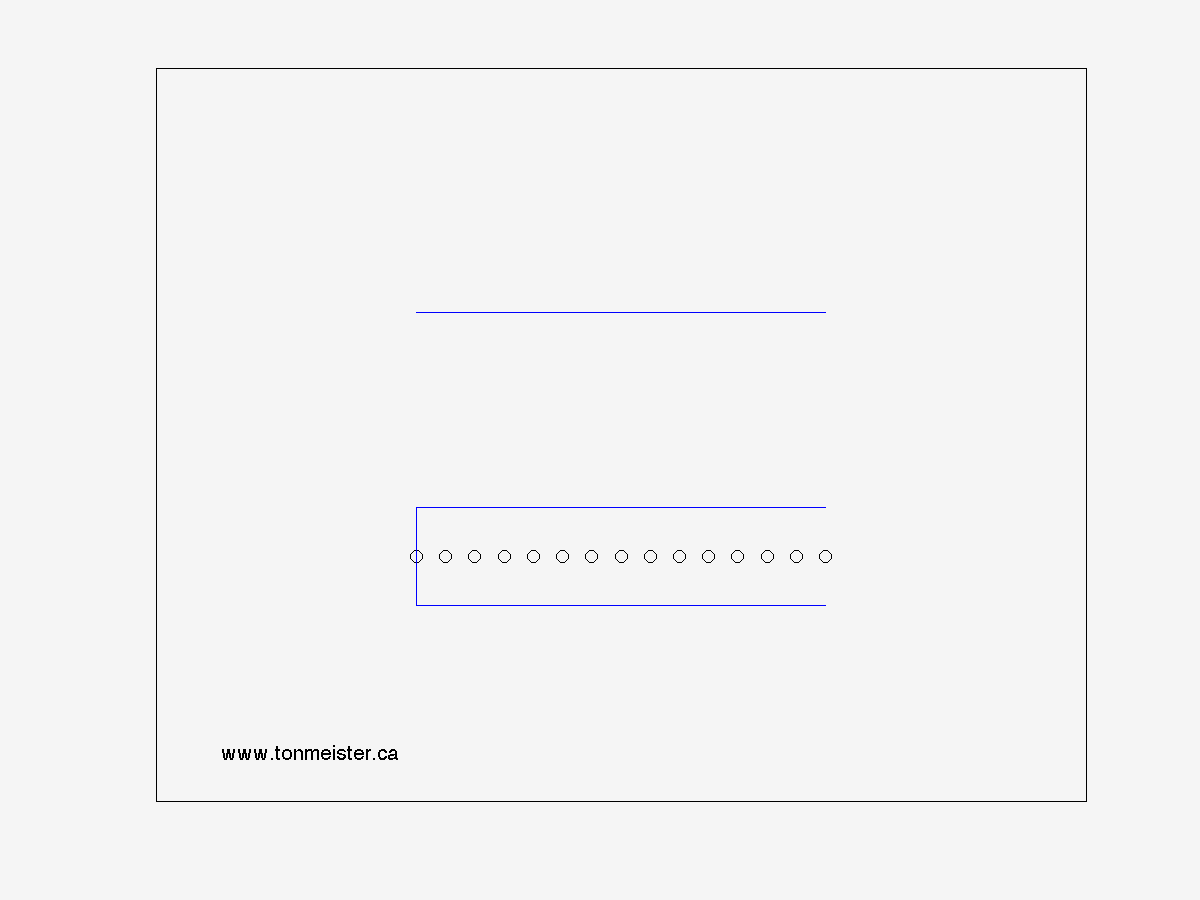Closed-Open Pipe

The first harmonic (also known as the fundamental) can be seen in Figure #1 below. The top plot with the red line is the graph typically seen in textbooks. It shows, in any frame, the instantaneous displacement of the air particles in the closed-open pipe (shown as circles in the lower plot) relative to their "resting" position. When the red line is positive (higher), the air particles have moved to the right. When the red line is negative (lower) the air particles have moved to the left.

Notice that the the red line is clamped to an immovable object on one end and free to move on the other (therefore it has one "high impedance termination" and one "low impedance termination"). Similarly, there is a cap on one end of the pipe (a high impedance) and no cap on the other end (a low impedance). This means that the last air particles at one end of the pipe (the capped end) can't move, and the particles at the other end are free to move in and out of the pipe.

Notice as well that the resonance has a wavelength equal to four times the length of the pipe. This is more easily seen in the red-lined plot than the drawings of the particles in the pipe.

One important thing to note here is that, typically, in textbooks, you'll see a plot that shows either the pressure or the velocity of the air particles. The red lines shown here are neither of these. As I mentioned above, they're the displacement of the air particles.Figure 1 - Harmonic #1

The second harmonic (shown below) occurs when the length of the pipe equals 75% of the wavelength of the resonance. This means that we have a point in the pipe where the air particles (and the string) don't move in addition to the immovable particles at the capped end. Note that the frequency of this resonance is 3 times the fundamental frequency (not 2 times, as in the case of the closed-closed and open-open pipes).Figure 2 - Harmonic #3

The third harmonic (shown below) occurs when the length of the pipe equals 1.25 times the wavelength of the resonance. This means that we have two points in the pipe where the air particles (and the string) don't move (apart from the capped end). Note that the frequency of this resonance is 5 times the fundamental frequency.Figure 3 - Harmonic #5Figure 4 - Harmonic #7